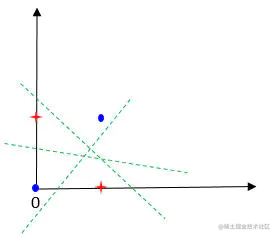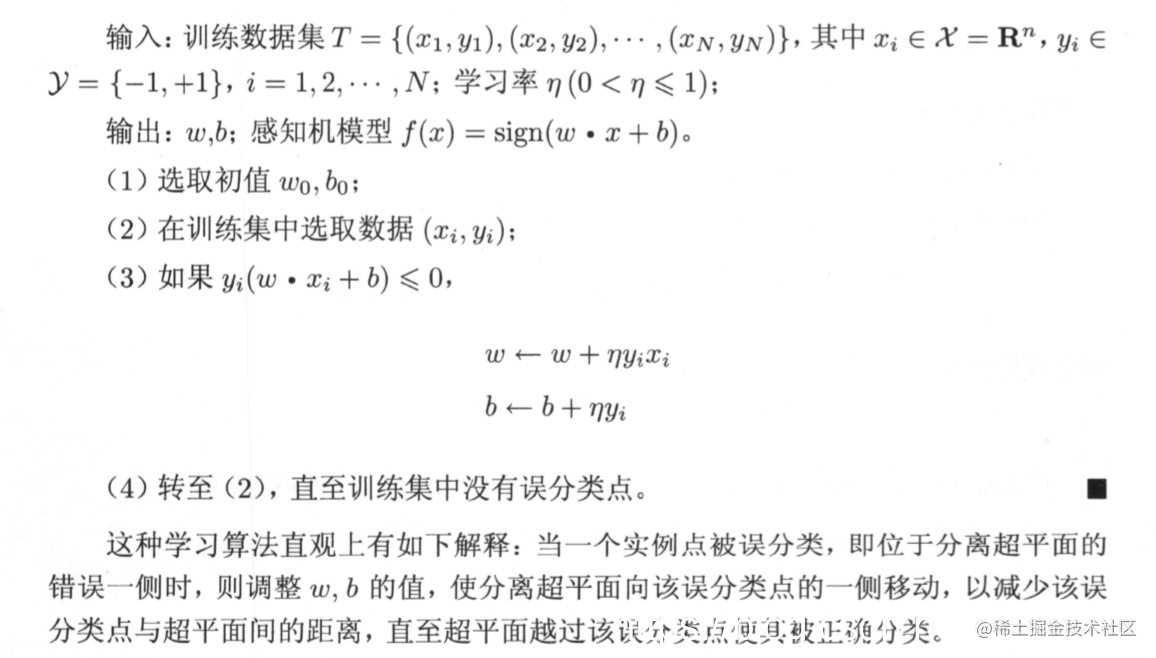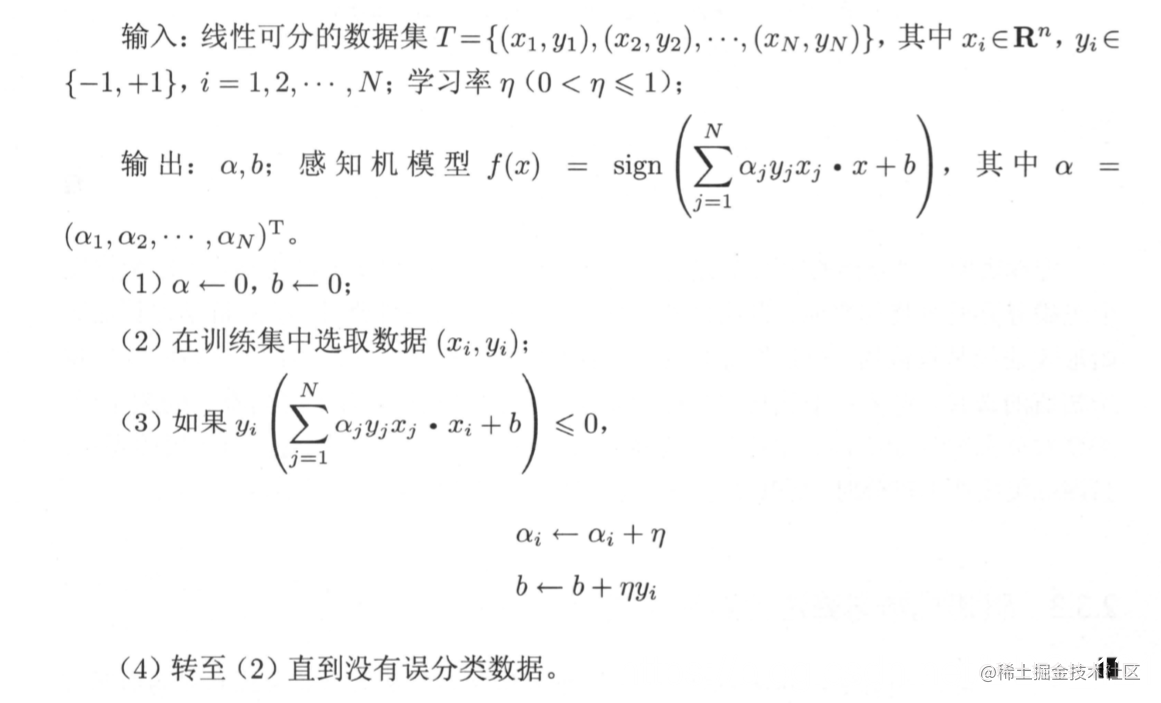# 统计学习方法-感知机概括和补充

## 前言

《统计学习方法》第二版出了有段时间了，最近得空可以拜读一下。之前看第一版的时候还是一年多以前，那个时候看的懵懵懂懂的，很吃力。希望这一次能够有所收获，能够收获新的东西，这些文章只是用于记录相关的一些要点，以及补充一下内容，仅供学习用。

### 第二章节的目录

• 感知机模型

• 感知机学习策略

• 数据集的线性可分性
• 感知机学习策略
• 感知机学习算法
• 感知机学习算法

• 感知机学习算法的原始形式
• 算法的收敛性
• 感知机学习算法的对偶形式

### 零零碎碎的一些记录

• 损失函数 L ( w , b ) L(w,b) L(w,b)的经验风险最小化
• 本章中涉及到向量内积，有超平面的概念，也有线性可分数据集的说明，在策略部分有说明损关于失函数的选择的考虑。另外，感知机和SVM的更多联系源自margin的思想， 实际上在本章的介绍中并没有体现margin的思想，参考文献中有给出对应的文献。
• 本章涉及的两个例子，思考一下为什么 η = 1 ​ \eta=1​ η=1​，进而思考一下参数空间，这两个例子设计了相应的测试案例实现， 在后面的内容中也有展示。
• 第一次涉及Gram Matrix G = [ x i ⋅ x j ] N × N G=[x_i\cdot x_j]_{N\times N} G=[xi​⋅xj​]N×N​
• 感知机的激活函数是符号函数。
• 感知机是神经网络和支持向量机的基础。
• 当我们讨论决策边界的时候, 实际上是在考虑算法的几何解释
• 关于感知机为什么不能处理异或问题, 可以借助下图理解。• 书中有提到函数间隔，几何间隔，这里间隔就是margin
• 分离超平面将特征空间划分为两个部分，一部分是正类， 一部分是负类。法向量指向的一侧为正类，另一侧为负类。
• 感知机损失函数 L = max ⁡ ( 0 , − y i ( w ⋅ x i + b ) ) L=\max(0, -y_i(w\cdot x_i+b)) L=max(0,−yi​(w⋅xi​+b))。

## 三要素

### 模型

• 输入空间： X ⊆ R n \mathcal X\sube \bf R^n X⊆Rn
• 输出空间： Y = + 1 , − 1 \mathcal Y={+1,-1} Y=+1,−1
• 决策函数： f ( x ) = s i g n ( w ⋅ x + b ) f(x)=sign (w\cdot x+b) f(x)=sign(w⋅x+b)

### 策略

• 确定学习策略就是定义(经验)损失函数并将损失函数最小化。
• 注意这里提到了经验，所以学习是base在训练数据集上的操作

#### 损失函数选择

• 损失函数的一个自然选择是误分类点的总数，但是，这样的损失函数不是参数 w , b w,b w,b的连续可导函数，不易优化
• 损失函数的另一个选择是误分类点到超平面 S S S的总距离，这是感知机所采用的感知机学习的经验风险函数(损失函数)
L ( w , b ) = − ∑ x i ∈ M y i ( w ⋅ x i + b ) L(w,b)=-\sum_{x_i\in M}y_i(w\cdot x_i+b) L(w,b)=−xi​∈M∑​yi​(w⋅xi​+b)
其中 M M M是误分类点的集合。给定训练数据集 T T T，损失函数 L ( w , b ) L(w,b) L(w,b)是 w w w和 b b b的连续可导函数

### 算法

#### 原始形式#### 对偶形式Gram matrix

G = [ x i ⋅ x j ] N × N G=[x_i\cdot x_j]_{N\times N} G=[xi​⋅xj​]N×N​

## 例子

### 例2.1

感知机学习算法由于采用不同的初值或选取不同的误分类点，解可以不同。

### 例2.2

• η = 1 \eta=1 η=1
• α i ← α i + 1 , b ← b + y i \alpha_i\leftarrow \alpha_i+1, b\leftarrow b+y_i αi​←αi​+1,b←b+yi​

• 为什么 η \eta η选了1，这样得到的值数量级是1
• 这个表达式中用到了上面的 η = 1 \eta=1 η=1这个结果，已经做了简化

## 问题

### 损失函数

感知机中的损失函数中的分母为什么可以不考虑？有些人解释是正数，不影响，但是分母中含有 w，而其也是未知数，在考虑损失函数的最值时候会不影响么？想不通

• 感知机处理线性可分数据集，二分类， Y = { + 1 , − 1 } \bf Y=\{+1,-1\} Y={+1,−1} ，所以涉及到的乘以的操作实际贡献的是符号；

• 损失函数 L ( w , b ) = − ∑ x i ∈ M y i ( w ⋅ x i + b ) L(w,b)=-\sum_{x_i\in M}y_i(w\cdot x_i+b) L(w,b)=−∑xi​∈M​yi​(w⋅xi​+b),其中 M M M 是错分的点集合，线性可分的数据集肯定能找到超平面 S S S， 所以这个损失函数最值是0。

• 如果正确分类， y i ( w ⋅ x i + b ) = ∣ w ⋅ x i + b ∣ y_i(w\cdot x_i+b)=|w\cdot x_i+b| yi​(w⋅xi​+b)=∣w⋅xi​+b∣ ，错误分类的话，为了保证正数就加个负号，这就是损失函数里面那个负号，这个就是函数间隔；

• 1 ∣ ∣ w ∣ ∣ \frac{1}{||w||} ∣∣w∣∣1​ 用来归一化超平面法向量，得到几何间隔，也就是点到超平面的距离， 函数间隔和几何间隔的差异在于同一个超平面 ( w , b ) (w,b) (w,b) 参数等比例放大成 ( k w , k b ) (kw,kb) (kw,kb) 之后，虽然表示的同一个超平面，但是点到超平面的函数间隔也放大了，但是几何间隔是不变的；

• 具体算法实现的时候， w ​ w​ w​要初始化，然后每次迭代针对错分点进行调整，既然要初始化，那如果初始化个 ∣ ∣ w ∣ ∣ = 1 ​ ||w||=1​ ∣∣w∣∣=1​ 的情况也就不用纠结了，和不考虑 1 ∣ ∣ w ∣ ∣ ​ \frac{1}{||w||}​ ∣∣w∣∣1​​ 是一样的了；

• 针对错分点是这么调整的

w ← w + η y i x i b ← b + η y i \begin{aligned} w&\leftarrow w+\eta y_ix_i\\ b&\leftarrow b+\eta y_i \end{aligned} wb​←w+ηyi​xi​←b+ηyi​​

• 感知机的解不唯一，和初值有关系，和误分类点调整顺序也有关系；
• 这么调整就能找到感知机的解？能，Novikoff还证明了，通过有限次搜索能找到将训练数据完全正确分开的分离超平面。